• 全国通用版2018_2019高中数学第二章平面向量2.3平面向量的数量2.3.3向量数量坐标运算与度量公式练习新人教B版必修4
• 2019_2020学年高中数学第2章平面向量2.3.3向量数量坐标运算与度量公式练习新人教B版必修4
• （新课程）2013高中数学 向量数量坐标运算与度量公式 课件 苏教版必修4
• 2015_2016学年高中数学2.3.3向量数量坐标运算与度量公式课时作业新人教B版必修4
• 辽宁省大连市理工大学附属高中数学 向量数量坐标运算与度量公式学案 新人教B版必修4
• ## 向量内积和基变换

千次阅读 2018-08-23 10:48:33
向量B的模为1，则A与B的内积值等于A向B所在直线投影的矢量长度 其实就是将B看成是一个基。 比如说原来在（0，1），（1，0）这个基下，（2，3）。 要求在这一组基的坐标，就可以直接内积 写成矩阵的形式就是： ...
参考： http://blog.codinglabs.org/articles/pca-tutorial.html
引用例子
A⋅B=|A|cos(a)  设向量B的模为1，则A与B的内积值等于A向B所在直线投影的矢量长度  其实就是将B看成是一个基。  比如说原来在（0，1），（1，0）这个基下，（2，3）。  要求在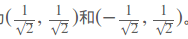这一组基的坐标，就可以直接内积  写成矩阵的形式就是：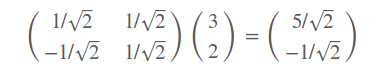数学表达
一般的，如果我们有M个N维向量，想将其变换为由R个N维向量表示的新空间中，那么首先将R个基按行组成矩阵A，然后将向量按列组成矩阵B，那么两矩阵的乘积AB就是变换结果，其中AB的第m列为A中第m列变换后的结果。

⎛⎝⎜⎜⎜⎜p11p21⋮pR1………p1Np2N⋮pRN⎞⎠⎟⎟⎟⎟R×N⎛⎝⎜⎜⎜⎜a11a12⋮a1N………aM1aM2⋮pMN⎞⎠⎟⎟⎟⎟N×M≜⎛⎝⎜⎜⎜⎜p1p2⋮pR⎞⎠⎟⎟⎟⎟(a1a2…aM)=⎛⎝⎜⎜⎜⎜p1a1p2a1⋮pRa1p1a2p2a2⋮pRa2……⋱…p1aMp2aM⋮pRaM⎞⎠⎟⎟⎟⎟R×M

(

p

11

…

p

1

N

p

21

…

p

2

N

⋮

⋮

p

R

1

…

p

R

N

)

R

×

N

(

a

11

…

a

M

1

a

12

…

a

M

2

⋮

⋮

a

1

N

…

p

M

N

)

N

×

M

≜

(

p

1

p

2

⋮

p

R

)

(

a

1

a

2

…

a

M

)

=

(

p

1

a

1

p

1

a

2

…

p

1

a

M

p

2

a

1

p

2

a

2

…

p

2

a

M

⋮

⋮

⋱

⋮

p

R

a

1

p

R

a

2

…

p

R

a

M

)

R

×

M

$\begin{pmatrix} p_{11} & \dots & p_{1N} \\ p_{21} & \dots & p_{2N} \\ \vdots & & \vdots \\ p_{R1} & \dots & p_{RN}\\ \end{pmatrix}_{R\times N} \begin{pmatrix} a_{11} & \dots & a_{M1} \\ a_{12} & \dots & a_{M2} \\ \vdots & & \vdots \\ a_{1N} & \dots & p_{MN}\\ \end{pmatrix}_{N\times M} \triangleq \begin{pmatrix} p_{1} \\ p_{2} \\ \vdots \\ p_{R} \end{pmatrix} \begin{pmatrix} a_{1} & a_{2} & \dots & a_{M} \end{pmatrix} =\begin{pmatrix} p_{1}a_{1} & p_{1}a_{2} & \dots & p_{1}a_{M} \\ p_{2}a_{1} & p_{2}a_{2} & \dots & p_{2}a_{M} \\ \vdots & \vdots & \ddots & \vdots \\ p_{R}a_{1} & p_{R}a_{2} & \dots & p_{R}a_{M} \end{pmatrix}_{R\times M}$
第一个矩阵R个N维向量的基，按行看第二个矩阵M个N维向量，按列看，表示第M个数据两个矩阵相乘的结果：
列解释：  和第二个矩阵对应，对应的列就是a矩阵在p这个基矩阵下的坐标。元素解释：  比如

p1a1

p

1

a

1

$p_{1}a_{1}$表示的是

a1

a

1

$a_{1}$在

p1

p

1

$p_{1}$这个基方向上的矢量投影
结论
两个矩阵相乘的意义是将右边矩阵中的每一列列向量变换到左边矩阵中每一行行向量为基所表示的空间中去R是可以小于N的，这样也就实现了降低维度,原来是a矩阵是M个N维的，现在通过R个N维的基矩阵，变成了M个R维
也就是说如果基的数量少于向量本身的维数，则可以达到降维的效果。基的个数可以决定降维后数据的维度。
展开全文降维 线性变换 基变换
• 向量是由n个实数组成的一个n行1列（n*1）或...向量的点乘,也叫向量内积、数量积，对两个向量执行点乘运算，就是对这两个向量对应位一一相乘之后求和的操作，点乘的结果是一个标量。 点乘公式 对于向量a和向量b：

向量是由n个实数组成的一个n行1列（n*1）或一个1行n列（1*n）的有序数组；

向量的点乘,也叫向量的内积、数量积，对两个向量执行点乘运算，就是对这两个向量对应位一一相乘之后求和的操作，点乘的结果是一个标量。

点乘公式

对于向量a和向量b：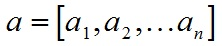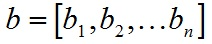a和b的点积公式为：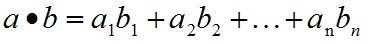要求一维向量a和向量b的行列数相同。

点乘几何意义

点乘的几何意义是可以用来表征或计算两个向量之间的夹角，以及在b向量在a向量方向上的投影，有公式：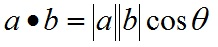推导过程如下，首先看一下向量组成：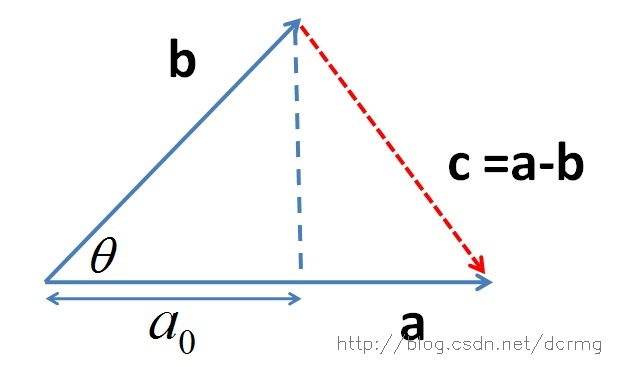定义向量：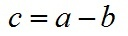根据三角形余弦定理有：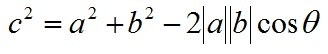根据关系c=a-b（a、b、c均为向量）有：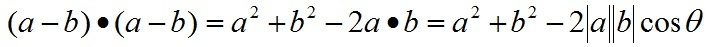即：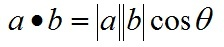向量a，b的长度都是可以计算的已知量，从而有a和b间的夹角θ：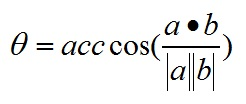根据这个公式就可以计算向量a和向量b之间的夹角。从而就可以进一步判断这两个向量是否是同一方向，是否正交(也就是垂直)等方向关系，具体对应关系为：
a·b>0    方向基本相同，夹角在0°到90°之间
a·b=0    正交，相互垂直
a·b<0    方向基本相反，夹角在90°到180°之间

叉乘公式

两个向量的叉乘，又叫向量积、外积、叉积，叉乘的运算结果是一个向量而不是一个标量。并且两个向量的叉积与这两个向量组成的坐标平面垂直。

对于向量a和向量b：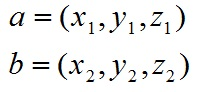a和b的叉乘公式为：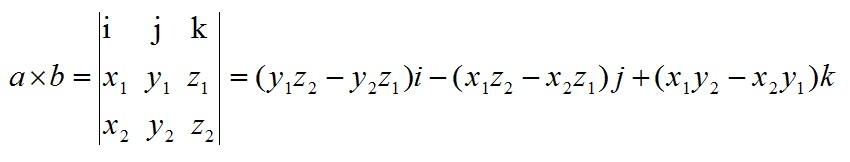其中：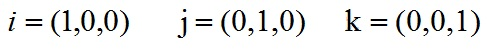根据i、j、k间关系，有：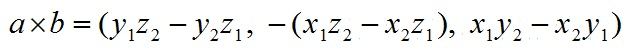叉乘几何意义

在三维几何中，向量a和向量b的叉乘结果是一个向量，更为熟知的叫法是法向量，该向量垂直于a和b向量构成的平面。

在3D图像学中，叉乘的概念非常有用，可以通过两个向量的叉乘，生成第三个垂直于a，b的法向量，从而构建X、Y、Z坐标系。如下图所示：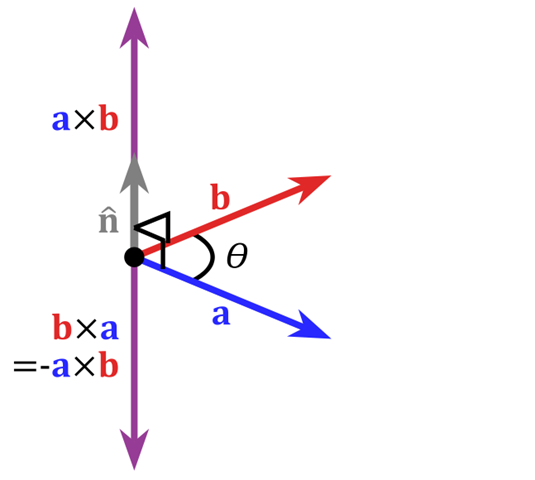在二维空间中，叉乘还有另外一个几何意义就是：aXb等于由向量a和向量b构成的平行四边形的面积。


展开全文• ## CDMA向量内积的计算

千次阅读 多人点赞 2020-03-03 14:02:39
CDMA向量内积的计算 在平面坐标上，有A点和B点，A点坐标是(x1, y1)，B点坐标是(x2, y2)。![图1](https://img-blog.csdnimg.cn/20200303134826109.png?x-oss-process=image/watermark,type_ZmFuZ3poZW5naGVpdGk,...
CDMA向量内积的计算
在平面坐标上，有A点和B点，A点坐标是

(

x

1

,

y

1

)

(x_{1}, y_{1})

，B点坐标是

(

x

2

,

y

2

)

(x_{2}, y_{2})

。图2

则

A

B

→

=

(

x

2

−

x

1

,

y

2

−

y

1

)

\overrightarrow{AB}=(x_{2}-x_{1},y_{2}-y_{1})

那么

A

B

→

\overrightarrow{AB}

向量的模是

∣

A

B

∣

=

(

x

2

−

x

1

)

2

+

(

y

2

−

y

1

)

2

\left|AB\right|=\sqrt{(x_{2}-x_{1})^2+(y_{2}-y_{1})^2}

即是线段AB的长度。 　　若A点在原点，即

x

1

=

0

x_{1}=0

，

y

1

=

0

y_{1}=0

，则

A

B

→

=

(

x

2

,

y

2

)

\overrightarrow{AB}=(x_{2},y_{2})

，如图2所示。图2

三维空间的向量就是在三维空间的两个点之间的带有方向和大小的量。在三维空间中有A和B点两，A点坐标是

(

x

1

,

y

1

,

z

1

)

(x_{1}, y_{1},z_{1})

，B点坐标是

(

x

2

,

y

2

,

z

2

)

(x_{2}, y_{2},z_{2})

。则

A

B

→

=

(

x

2

−

x

1

,

y

2

−

y

1

,

z

2

−

z

1

)

\overrightarrow{AB}=(x_{2}-x_{1},y_{2}-y_{1},z_{2}-z_{1})

其他同理。 　　如图3所示，在二维平面上有两个向量

ａ

⃗

=

(

a

1

,

a

2

)

\vec{ａ}=(a_{1},a_{2})

，

b

⃗

=

(

b

1

,

b

2

)

\vec{b}=(b_{1},b_{2})

，则内积

ａ

⃗

⋅

b

⃗

=

∣

ａ

⃗

∣

∣

b

⃗

∣

cos

⁡

θ

(

1

)图3

若

ａ

⃗

\vec{ａ}

，

b

⃗

\vec{b}

垂直，则

cos

⁡

θ

=

1

\cos\theta=1

则

ａ

⃗

⋅

b

⃗

=

∣

ａ

⃗

∣

∣

b

⃗

∣

cos

⁡

θ

=

∣

ａ

⃗

∣

∣

b

⃗

∣

(

2

)

由(1)式可得

ａ

⃗

⋅

b

⃗

=

a

1

b

1

+

a

2

b

2

(

3

)

例1
如图4所示，图中有两个向量

ａ

⃗

\vec{ａ}

与

b

⃗

\vec{b}

，A，B，C三点的坐标分别为A(1,2)，B(2,4)，C(3,1)。则

ａ

⃗

=

(

a

1

,

a

2

)

=

(

2

−

1

,

4

−

2

)

=

(

1

,

2

)

\vec{ａ}=(a_{1},a_{2})=(2-1,4-2)=(1,2)

b

⃗

=

(

b

1

,

b

2

)

=

(

3

−

1

,

1

−

2

)

=

(

2

,

−

1

)

\vec{b}=(b_{1},b_{2})=(3-1,1-2)=(2,-1)

所以

ａ

⃗

⋅

b

⃗

=

a

1

b

1

+

a

2

b

2

=

(

1

×

2

+

2

×

(

−

1

)

)

=

0

\vec{ａ} \cdot \vec{b}=a_{1}b_{1}+a_{2}b_{2}=(1×2+2×(-1))=0

因此，向量

a

⃗

\vec{a}

和

b

⃗

\vec{b}

正交，且两向量垂直。 规格化内积

ａ

⃗

⋅

b

⃗

=

1

2

(

a

1

b

1

+

a

2

b

2

)

\vec{ａ} \cdot \vec{b}=\frac12(a_{1}b_{1}+a_{2}b_{2})

而规格化内积

ａ

⃗

⋅

a

⃗

=

1

2

(

a

1

a

1

+

a

2

a

2

)

=

1

2

(

1

×

1

+

2

×

2

)

=

2.5

≠

1

\vec{ａ} \cdot \vec{a}=\frac12(a_{1}a_{1}+a_{2}a_{2})=\frac12(1×1+2×2)=2.5≠1

假设码片向量是2维的，这个2维的向量是不能作为发送站的码片向量的。图4

当两个m维向量有两个向量

ａ

⃗

=

(

a

1

,

a

2

,

⋯

a

m

)

\vec{ａ}=(a_{1},a_{2},{\cdots}a_{m})

，

b

⃗

=

(

b

1

,

b

2

,

⋯

b

m

)

\vec{b}=(b_{1},b_{2},{\cdots}b_{m})

，则规格化内积为

ａ

⃗

⋅

b

⃗

=

1

m

∑

i

=

0

m

a

i

b

i

=

1

m

(

a

1

b

1

+

a

2

b

2

+

⋯

+

a

m

b

m

)

(

4

)

例2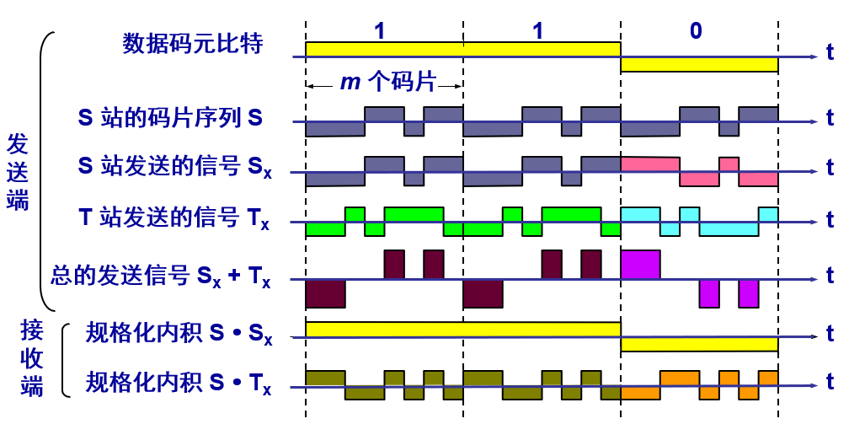S站的码片序列S是（-1 -1 -1 +1 +1 -1 +1 +1） T站的码片序列T是（-1 -1 +1 -1 +1 +1 +1 -1） 当数据码元比特为1时，发送信号

S

x

+

T

x

S_{x}+T_{x}

是（-2 -2 0 0 2 0 2 0） 因为

S

→

⋅

(

S

x

→

+

T

x

→

)

=

S

→

⋅

S

x

→

+

S

→

⋅

T

x

→

\overrightarrow{S}\cdot (\overrightarrow{S_{x}}+\overrightarrow{T_{x}})=\overrightarrow{S}\cdot \overrightarrow{S_{x}}+\overrightarrow{S}\cdot \overrightarrow{T_{x}}

且规格化内积

S

→

⋅

S

x

→

=

1

8

[

(

−

1

)

×

(

−

1

)

+

(

−

1

)

×

(

−

1

)

+

(

−

1

)

×

(

−

1

)

+

(

+

1

)

×

(

+

1

)

+

(

+

1

)

×

(

+

1

)

+

(

−

1

)

×

(

−

1

)

+

(

+

1

)

×

(

+

1

)

+

(

+

1

)

×

(

+

1

)

]

=

1

\overrightarrow{S}\cdot \overrightarrow{S_{x}}=\frac{1}{8}[(-1)×(-1)+(-1)×(-1)+(-1)×(-1)+(+1)×(+1)+ (+1)×(+1)+(-1)×(-1)+(+1)×(+1)+(+1)×(+1)]=1

规格化内积

S

→

⋅

T

x

→

=

1

8

[

(

−

1

)

×

(

−

1

)

+

(

−

1

)

×

(

−

1

)

+

(

−

1

)

×

(

+

1

)

+

(

+

1

)

×

(

−

1

)

+

(

+

1

)

×

(

+

1

)

+

(

−

1

)

×

(

+

1

)

+

(

+

1

)

×

(

+

1

)

+

(

+

1

)

×

(

−

1

)

]

=

0

\overrightarrow{S}\cdot \overrightarrow{T_{x}}=\frac{1}{8}[(-1)×(-1)+(-1)×(-1)+(-1)×(+1)+(+1)×(-1)+(+1)×(+1)+(-1)×(+1)+(+1)×(+1)+(+1)×(-1)]=0

所以

S

→

⋅

(

S

x

→

+

T

x

→

)

=

S

→

⋅

S

x

→

+

S

→

⋅

T

x

→

=

1

+

0

=

1

\overrightarrow{S}\cdot (\overrightarrow{S_{x}}+\overrightarrow{T_{x}})=\overrightarrow{S}\cdot \overrightarrow{S_{x}}+\overrightarrow{S}\cdot \overrightarrow{T_{x}}=1+0=1

所以S站发出的数据码元为1。 若计算的结果为-1，则说明S站发出的数据码元为0，若计算结果为0，则说明S站没有发送数据。
例3展开全文• 向量内积（点乘） 定义 概括地说，向量内积（点乘/数量积）。对两个向量执行点乘运算，就是对这两个向量对应位一一相乘之后求和的操作，如下所示，对于向量a和向量b： a和b的点积公式为： 这里要求一维...
向量的内积（点乘）
定义
概括地说，向量的内积（点乘/数量积）。对两个向量执行点乘运算，就是对这两个向量对应位一一相乘之后求和的操作，如下所示，对于向量a和向量b：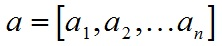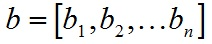a和b的点积公式为：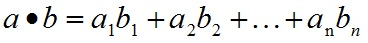这里要求一维向量a和向量b的行列数相同。注意：点乘的结果是一个标量(数量而不是向量)
定义：两个向量a与b的内积为 a·b = |a||b|cos∠(a, b)，特别地，0·a =a·0 = 0；若a，b是非零向量，则a与b****正交的充要条件是a·b= 0。
向量内积的性质：
a^2 ≥ 0；当a^2 = 0时，必有a = 0. （正定性）a·b = b·a. （对称性）(λa + μb)·c = λa·c + μb·c，对任意实数λ, μ成立. （线性）cos∠(a,b) =a·b/(|a||b|).|a·b| ≤ |a||b|，等号只在a与b共线时成立.
向量内积的几何意义
内积（点乘）的几何意义包括：
表征或计算两个向量之间的夹角b向量在a向量方向上的投影
有公式：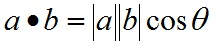推导过程如下，首先看一下向量组成：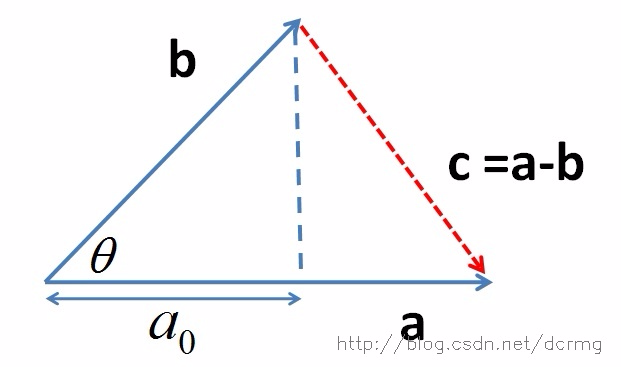定义向量c：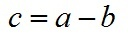根据三角形余弦定理（这里a、b、c均为向量，下同）有：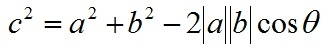根据关系c=a-b有：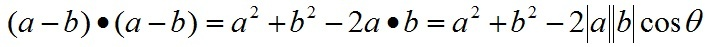即：
a∙b=|a||b|cos⁡(θ)
向量a，b的长度都是可以计算的已知量，从而有a和b间的夹角θ：
θ=arccos⁡(a∙b|a||b|)
进而可以进一步判断两个向量是否同一方向或正交(即垂直)等方向关系，具体对应关系为：
a∙b>0→方向基本相同，夹角在0°到90°之间 a∙b=0→ 正交，相互垂直 a∙b<0→ 方向基本相反，夹角在90°到180°之间
向量的外积（叉乘）
定义
概括地说，两个向量的外积，又叫叉乘、叉积向量积，其运算结果是一个向量而不是一个标量。并且两个向量的外积与这两个向量组成的坐标平面垂直。
定义：向量a与b的外积a×b是一个向量，其长度等于|a×b| = |a||b|sin∠(a,b)，其方向正交于a与b。并且，(a,b,a×b)构成右手系。 特别地，0×a = a×0 = 0.此外，对任意向量a，a×a=0。
对于向量a和向量b：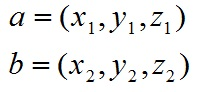a和b的外积公式为：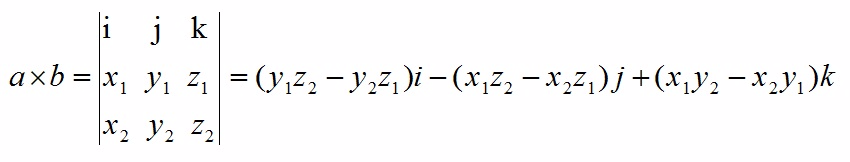其中：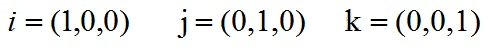根据i、j、k间关系，有：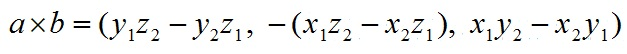向量外积的性质
a × b = -b × a. （反称性）(λa + μb) × c = λ(a ×c) + μ(b ×c). （线性）
向量外积的几何意义
在三维几何中，向量a和向量b的外积结果是一个向量，有个更通俗易懂的叫法是法向量，该向量垂直于a和b向量构成的平面。
在3D图像学中，外积的概念非常有用，可以通过两个向量的外积，生成第三个垂直于a，b的法向量，从而构建X、Y、Z坐标系。如下图所示：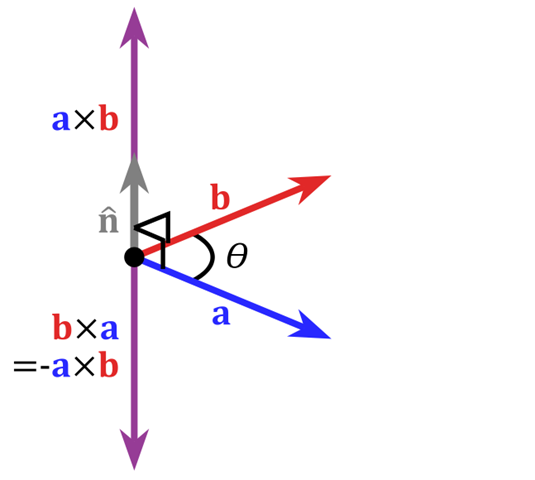在二维空间中，外积还有另外一个几何意义就是：|a×b|在数值上等于由向量a和向量b构成的平行四边形的面积。
Reference
http://blog.csdn.net/dcrmg/article/details/52416832http://blog.csdn.net/baimafujinji/article/details/50237081http://blog.csdn.net/hunter_wwq/article/details/41248537
展开全文• 对于运动学逆解或者姿态求解过程中存在的矩阵的计算的一部分内容点，斜对称阵，叉乘
• 向量内积（点乘） 定义 概括地说，向量内积（点乘/数量积）。对两个向量执行点乘运算，就是对这两个向量对应位一一相乘之后求和的操作，如下所示，对于向量a和向量b： a和b的点积公式为： 这里要求一维...
• 向量内积（点乘） 定义 概括地说，向量内积（点乘/数量积）。对两个向量执行点乘运算，就是对这两个向量对应位一一相乘之后求和的操作，如下所示，对于向量a和向量b： a和b的点积公式为： 这里要求一维...
• ## 向量的内积与外积

千次阅读 2019-11-11 16:35:36
向量内积与外积 向量内积 已知两个非零向量a、b，那么|a||b|cosθ（θ是a与b的夹角）叫做a与b的数量积或内积。记作a·b。两个向量的数量积等于它们对应坐标的乘积的和。即：若a=(x1,y1),b=(x2,y2)，则a·b=x1·...
• 参考： https://blog.csdn.net/dcrmg/article/details/52416832 ... 1 向量内积（点乘） 公式 a和b的点积（点乘）公式为： 向量内积的几何意义及用途 包括： 表征或计算两个向量之间的夹角 b向量在a向量...
• ## 向量的内积和外积

千次阅读 2019-11-11 19:27:55
向量的点乘,也叫向量内积、数量积，对两个向量执行点乘运算，就是对这两个向量对应位一一相乘之后求和的操作，点乘的结果是一个标量。 点乘公式 对于向量a和向量b： a=[a1,a2,a3,…,an]a=[a_1,a_2,a_3,…,a_n]a=...点乘和叉乘
• 一个行向量乘以一个列向量称作向量内积，又叫作点积，结果是一个数； 一个列向量乘以一个行向量称作向量的外积，外积是一种特殊的克罗内克积，结果是一个矩阵， 假设和b分别是一个行向量和一个列向量，那么内积...
• 向量是由n个实数组成的一个n行1列（n*1）或一个1行n列（1*n）的有序数组；...向量的点乘,也叫向量内积、数量积，对两个向量执行点乘运算，就是对这两个向量对应位一一相乘之后求和的操作，点...点乘
• 内积 计算公式 几何意义 两个向量之间的夹角 向量b在向量a上的投影 推导过程 外积 计算公式 几何意义 两个向量构成的平面的法向量 构件三维坐标系 外积在数值上等于两个向量组成平行四边形的面积 ...
• 向量 a⃗=(x1,y1)，b⃗=(x2,y2)\vec a=(x_1,y_1)，\vec b=(x_2,y_2)a=(x1​,y1​)，b=(x2​,y2​)，夹角为 θ\thetaθ，内积为： a⃗⋅b⃗=∣a⃗∣∣b⃗∣cos⁡θ=x1x2+y1y2 \vec a \cdot \vec b=|\vec a||\vec b|...线性代数
• 向量的点乘,也叫向量内积、数量积，对两个向量执行点乘运算，就是对这两个向量对应位一一相乘之后求和的操作，点乘的结果是一个标量。 点乘公式 对于向量a和向量b： &nbsp; &nbsp; &nbsp; &...点乘
• 1.向量点乘公式推导和几何解释一般来说，点乘结果描述了两个向量的“相似”程度，点乘结果越大，两向量越相近。01.向量点乘(dot product)是其各个分量乘积的和，公式：用连加号写：02.几何解释：点乘的结果是一个...线性代数
• 向量内积（点乘）与外积（叉乘） 向量内积=点乘 向量的外积=叉乘 向量内积（点乘） 内积的几何意义： 用来表征或计算两个向量之间的夹角 在b向量在a向量方向上的投影。 向量的外积（叉乘） 两个向量的外...线性代数 机器学习
• 点在直线的投影坐标 n维向量投影坐标 几何投影坐标 一、点在直线的投影坐标  如下图所示，直线l1：y=kx+b，直线外有一点P(x0, y0)，问：点P在直线上的投影坐标为多少呢？   求点P的投影坐标，即是求过点P(x0,......